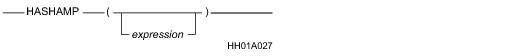## SQL Functions, Operators, Expressions, and Predicates

Product
Release Number
15.00
Language
English (United States)
Last Update
2018-09-24
dita:id
B035-1145
lifecycle
previous
Product Category

## HASHAMP

### Purpose

Returns the identification number of the primary AMP corresponding to the specified hash bucket number. If no hash bucket number is specified, HASHAMP returns one less than the maximum number of AMPs in the system.

### Syntaxwhere:

 Syntax element … Specifies … expression an optional expression that evaluates to a valid hash bucket number. For information on obtaining a hash bucket number that you can use for expression, see “HASHBUCKET” on page 898.

### ANSI Compliance

This is a Teradata extension to the ANSI SQL:2011 standard.

### Argument Type and Value

The expression argument must evaluate to INTEGER data type where the valid range of values depends on the system setting for the hash bucket size.

 IF the hash bucket size is … THEN the range of values for expression is … 16 bits 0 to 65535. 20 bits 0 to 1048575.

For information on how to specify the system setting for the hash bucket size, see “DBS Control utility” in Utilities: Volume 1 (A-K).

If expression cannot be implicitly converted to an INTEGER, an error is reported.

If expression results in a UDT, Teradata Database performs implicit type conversion on the UDT, provided that the UDT has an implicit cast that casts between the UDT and any of the following predefined types:

• Numeric
• Character
• DATE
• To define an implicit cast for a UDT, use the CREATE CAST statement and specify the AS ASSIGNMENT clause. For more information on CREATE CAST, see SQL Data Definition Language.

Implicit type conversion of UDTs for system operators and functions, including HASHAMP, is a Teradata extension to the ANSI SQL standard. To disable this extension, set the DisableUDTImplCastForSysFuncOp field of the DBS Control Record to TRUE. For details, see Utilities: Volume 1 (A-K).

For more information on implicit type conversion, see Chapter 13: “Data Type Conversions.”

### Result

 IF expression … THEN … evaluates to a valid hash bucket number HASHAMP determines the primary AMP corresponding to the hash bucket and returns the AMP identification number. The result is an INTEGER value that is greater than or equal to zero and less than the maximum number of AMPs in the configuration. does not appear in the argument list HASHAMP returns an INTEGER value that is one less than the maximum number of AMPs in the system. evaluates to NULL HASHAMP returns NULL.

For information on the hash map that defines the relationship between hash buckets and primary AMPs, see “Reconfiguration utility” Utilities: Volume 2 (L-Z).

### Examples

The following examples assume a table T with columns column_1, column_2, and an INTEGER column B populated with integer numbers from zero to the maximum number of hash buckets on the system.

`   CREATE TABLE T`
`      (column_1 INTEGER`
`      ,column_2 INTEGER`
`      ,B INTEGER)`
`   UNIQUE PRIMARY INDEX (column_1, column_2);`

### Example

If you call HASHAMP without an argument, it returns one less than the maximum number of AMPs on the system.

`   SELECT HASHAMP();`

### Example

If you call HASHAMP with an argument of NULL, it returns NULL.

`   SELECT HASHAMP(NULL);`

### Example

The following query returns the distribution of the hash buckets among the primary AMPs.

`   SELECT B, HASHAMP (B)`
`   FROM T`
`   ORDER BY 1;`

### Example

The following query returns the number of rows on each primary AMP where column_1 and column_2 are to be the primary index of table T.

`   SELECT HASHAMP (HASHBUCKET (HASHROW (column_1,column_2))), COUNT (*)`
`   FROM T`
`   GROUP BY 1`
`   ORDER BY 1;`